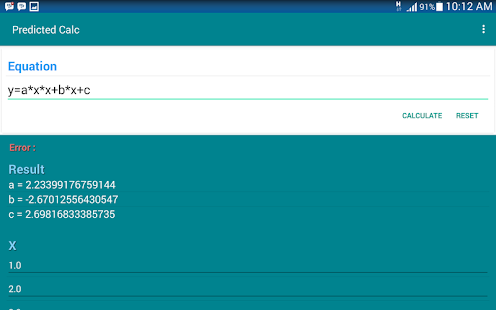## Posts

Showing posts from June, 2015

### Genetics Algorithm Method with Progressive Error PredictionGenetics Algorithm is one of approximation method that used base prediction technique. It's suggest the algorithm that can be used as portable finding method to approach unknown value whatever mathematics problem that given base on fitness value that can be reach. Neural Network have also capability as same as genetic algorithm. but it's have different approximation method where weight value is a sign that the best prediction can be reach.
Progressive error prediction is a method to implement Genetic Algorithm for fast finding algorithm. The idea is came from the numerical calculation with ordinary numerical approximation oftentimes given the infinite results value, if the case solver that implemented to the calculation not suitable as expectation, calculation will be stuck and can't be continued. With implement progressive error prediction, the random prediction can be directed to the right point and avoiding stuck point, so the calculation still will be continued alth…